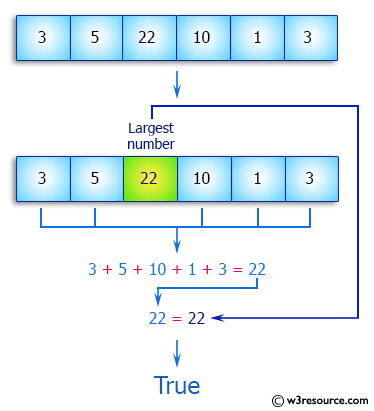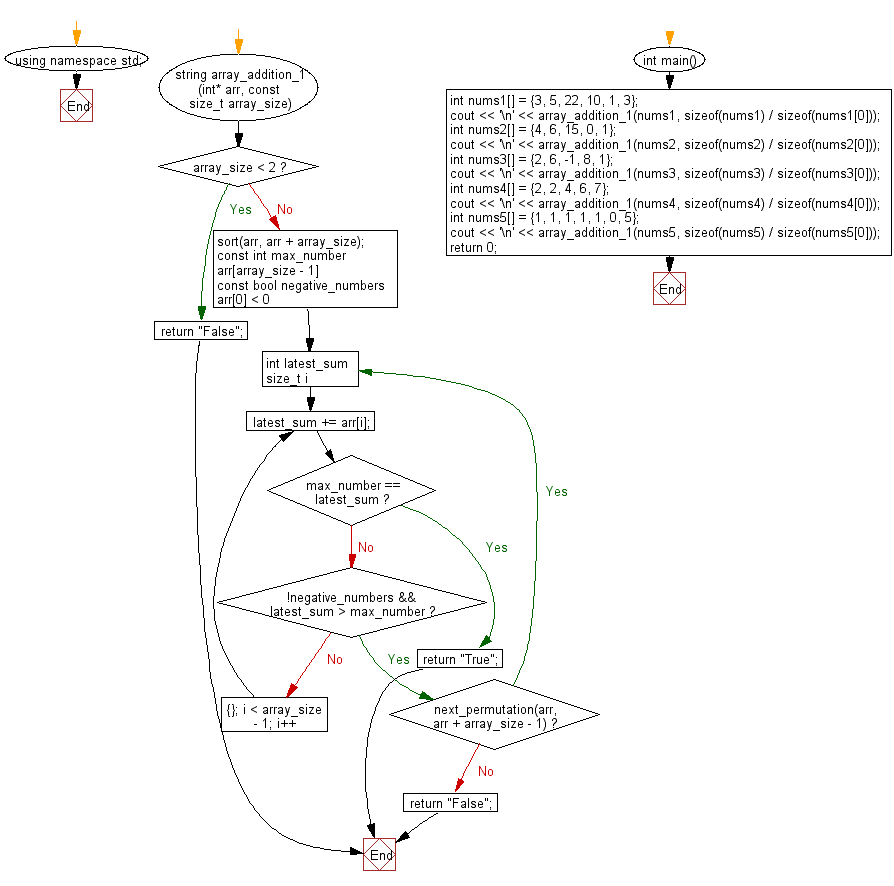﻿ C++ : Sum up some numbers in an array to get the largest# C++ Exercises: Arrange the numbers of a given array in a way that the sum of some numbers equal the largest number in the array

## C++ Array: Exercise-28 with Solution

Write a C++ program to arrange the numbers of a given array in a way that the sum of some numbers equal the largest number in the array.

Pictorial Presentation:Sample Solution:

C++ Code :

``````#include <iostream>
#include <algorithm>
#include <string>

using namespace std;

string array_addition_1(int* arr, const size_t array_size) {
if (array_size < 2)
return "False";

sort(arr, arr + array_size);

const int max_number{arr[array_size - 1]};

const bool negative_numbers{arr < 0};

do {
int latest_sum{};

for (size_t i{}; i < array_size - 1; i++) {
latest_sum += arr[i];

if (max_number == latest_sum)
return "True";
if (!negative_numbers && latest_sum > max_number)
break;
}

} while (next_permutation(arr, arr + array_size - 1));

return "False";
}

int main() {
int nums1[] = {3, 5, 22, 10, 1, 3};
cout << '\n' << array_addition_1(nums1, sizeof(nums1) / sizeof(nums1)); // "true" 3 + 5 + 10 + 1 + 3 = 22
int nums2[] = {4, 6, 15, 0, 1};
cout << '\n' << array_addition_1(nums2, sizeof(nums2) / sizeof(nums2)); //  "false"
int nums3[] = {2, 6, -1, 8, 1};
cout << '\n' << array_addition_1(nums3, sizeof(nums3) / sizeof(nums3)); // "true" 2 + 6 - 1 + 1 = 8
int nums4[] = {2, 2, 4, 6, 7};
cout << '\n' << array_addition_1(nums4, sizeof(nums4) / sizeof(nums4));  // "false"
int nums5[] = {1, 1, 1, 1, 1, 0, 5};
cout << '\n' << array_addition_1(nums5, sizeof(nums5) / sizeof(nums5)); // "true" 1 + 1 + 1 + 1 + 1 = 5
return 0;
}
``````

Sample Output:

```True
False
True
False
True
```

Flowchart:C++ Code Editor:

What is the difficulty level of this exercise?

Test your Programming skills with w3resource's quiz.

﻿